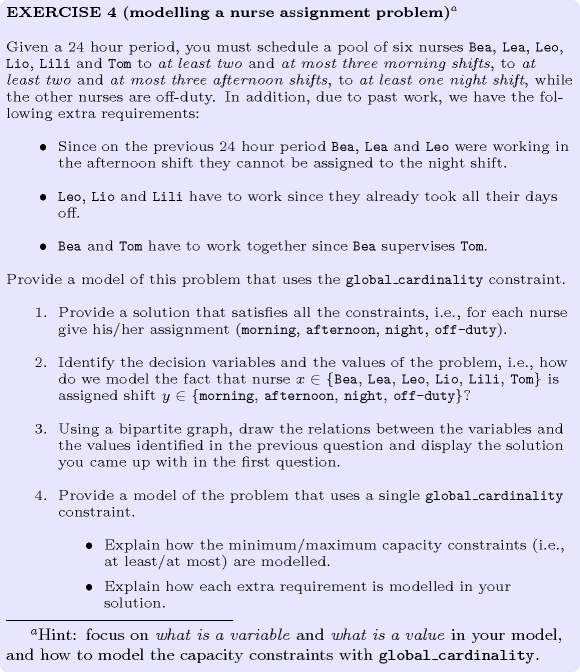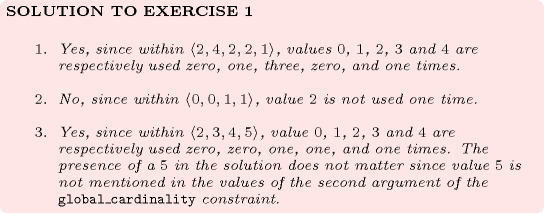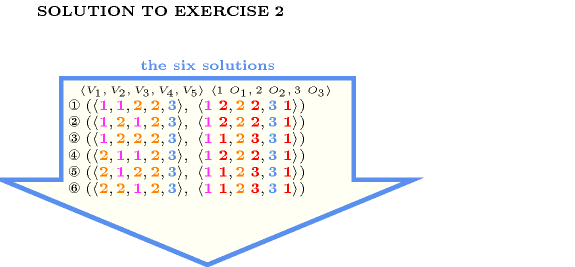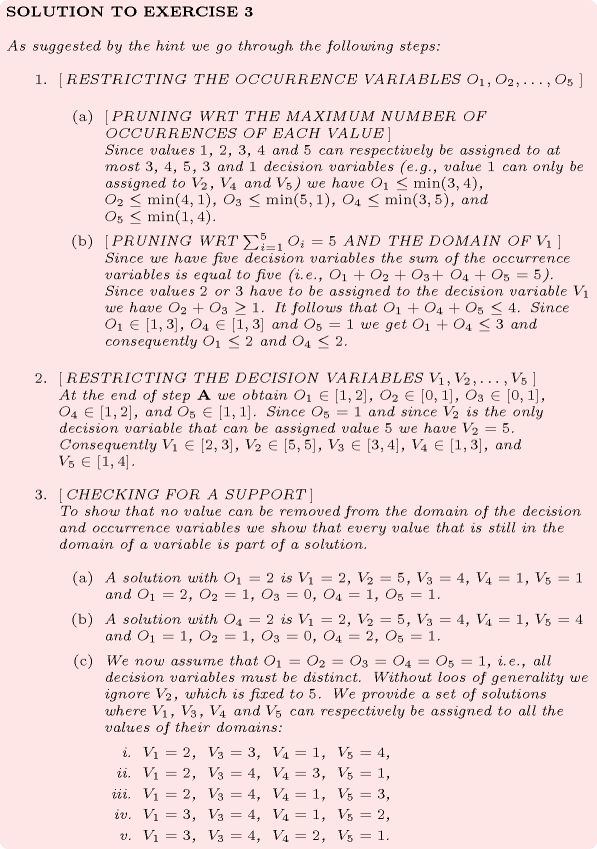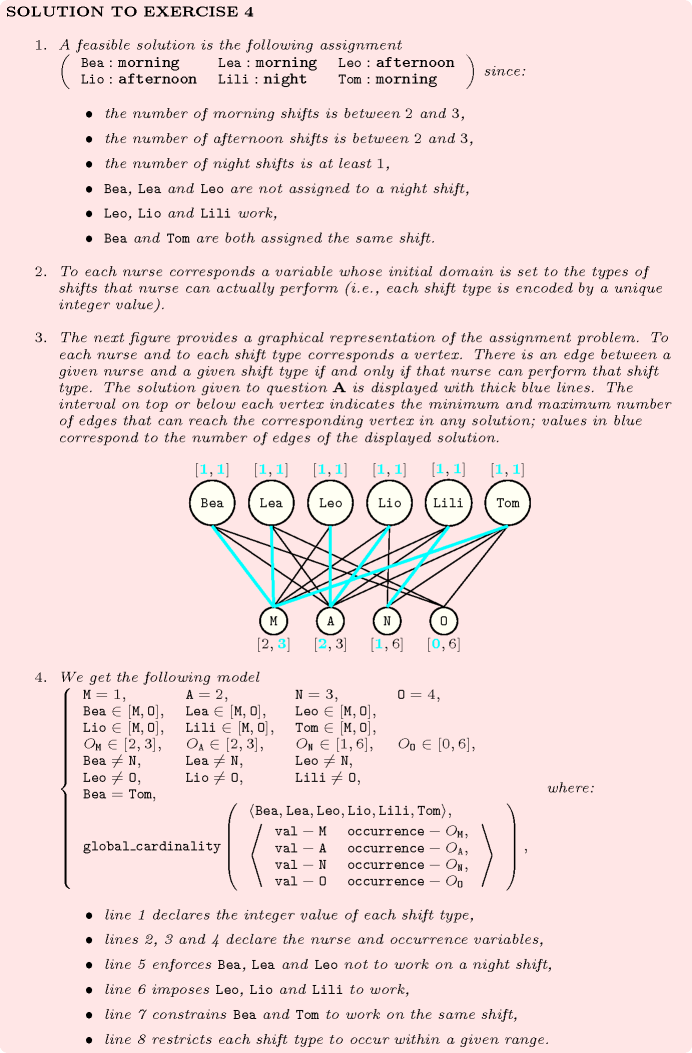## 5.163. global_cardinality

Origin
Constraint

$\mathrm{𝚐𝚕𝚘𝚋𝚊𝚕}_\mathrm{𝚌𝚊𝚛𝚍𝚒𝚗𝚊𝚕𝚒𝚝𝚢}\left(\mathrm{𝚅𝙰𝚁𝙸𝙰𝙱𝙻𝙴𝚂},\mathrm{𝚅𝙰𝙻𝚄𝙴𝚂}\right)$

Synonyms

$\mathrm{𝚌𝚘𝚞𝚗𝚝}$, $\mathrm{𝚍𝚒𝚜𝚝𝚛𝚒𝚋𝚞𝚝𝚎}$, $\mathrm{𝚍𝚒𝚜𝚝𝚛𝚒𝚋𝚞𝚝𝚒𝚘𝚗}$, $\mathrm{𝚐𝚌𝚌}$, $\mathrm{𝚌𝚊𝚛𝚍}_\mathrm{𝚟𝚊𝚛}_\mathrm{𝚐𝚌𝚌}$, $\mathrm{𝚎𝚐𝚌𝚌}$, $\mathrm{𝚎𝚡𝚝𝚎𝚗𝚍𝚎𝚍}_\mathrm{𝚐𝚕𝚘𝚋𝚊𝚕}_\mathrm{𝚌𝚊𝚛𝚍𝚒𝚗𝚊𝚕𝚒𝚝𝚢}$.

Arguments
 $\mathrm{𝚅𝙰𝚁𝙸𝙰𝙱𝙻𝙴𝚂}$ $\mathrm{𝚌𝚘𝚕𝚕𝚎𝚌𝚝𝚒𝚘𝚗}\left(\mathrm{𝚟𝚊𝚛}-\mathrm{𝚍𝚟𝚊𝚛}\right)$ $\mathrm{𝚅𝙰𝙻𝚄𝙴𝚂}$ $\mathrm{𝚌𝚘𝚕𝚕𝚎𝚌𝚝𝚒𝚘𝚗}\left(\mathrm{𝚟𝚊𝚕}-\mathrm{𝚒𝚗𝚝},\mathrm{𝚗𝚘𝚌𝚌𝚞𝚛𝚛𝚎𝚗𝚌𝚎}-\mathrm{𝚍𝚟𝚊𝚛}\right)$
Restrictions
 $\mathrm{𝚛𝚎𝚚𝚞𝚒𝚛𝚎𝚍}$$\left(\mathrm{𝚅𝙰𝚁𝙸𝙰𝙱𝙻𝙴𝚂},\mathrm{𝚟𝚊𝚛}\right)$ $\mathrm{𝚛𝚎𝚚𝚞𝚒𝚛𝚎𝚍}$$\left(\mathrm{𝚅𝙰𝙻𝚄𝙴𝚂},\left[\mathrm{𝚟𝚊𝚕},\mathrm{𝚗𝚘𝚌𝚌𝚞𝚛𝚛𝚎𝚗𝚌𝚎}\right]\right)$ $\mathrm{𝚍𝚒𝚜𝚝𝚒𝚗𝚌𝚝}$$\left(\mathrm{𝚅𝙰𝙻𝚄𝙴𝚂},\mathrm{𝚟𝚊𝚕}\right)$ $\mathrm{𝚅𝙰𝙻𝚄𝙴𝚂}.\mathrm{𝚗𝚘𝚌𝚌𝚞𝚛𝚛𝚎𝚗𝚌𝚎}\ge 0$ $\mathrm{𝚅𝙰𝙻𝚄𝙴𝚂}.\mathrm{𝚗𝚘𝚌𝚌𝚞𝚛𝚛𝚎𝚗𝚌𝚎}\le |\mathrm{𝚅𝙰𝚁𝙸𝙰𝙱𝙻𝙴𝚂}|$
Purpose

Each value $\mathrm{𝚅𝙰𝙻𝚄𝙴𝚂}\left[i\right].\mathrm{𝚟𝚊𝚕}$ (with $i\in \left[1,|\mathrm{𝚅𝙰𝙻𝚄𝙴𝚂}|\right]$) should be taken by exactly $\mathrm{𝚅𝙰𝙻𝚄𝙴𝚂}\left[i\right].\mathrm{𝚗𝚘𝚌𝚌𝚞𝚛𝚛𝚎𝚗𝚌𝚎}$ variables of the $\mathrm{𝚅𝙰𝚁𝙸𝙰𝙱𝙻𝙴𝚂}$ collection.

Example
$\left(\begin{array}{c}〈3,3,8,6〉,\hfill \\ 〈\begin{array}{cc}\mathrm{𝚟𝚊𝚕}-3\hfill & \mathrm{𝚗𝚘𝚌𝚌𝚞𝚛𝚛𝚎𝚗𝚌𝚎}-2,\hfill \\ \mathrm{𝚟𝚊𝚕}-5\hfill & \mathrm{𝚗𝚘𝚌𝚌𝚞𝚛𝚛𝚎𝚗𝚌𝚎}-0,\hfill \\ \mathrm{𝚟𝚊𝚕}-6\hfill & \mathrm{𝚗𝚘𝚌𝚌𝚞𝚛𝚛𝚎𝚗𝚌𝚎}-1\hfill \end{array}〉\hfill \end{array}\right)$

The $\mathrm{𝚐𝚕𝚘𝚋𝚊𝚕}_\mathrm{𝚌𝚊𝚛𝚍𝚒𝚗𝚊𝚕𝚒𝚝𝚢}$ constraint holds since values 3, 5 and 6 respectively occur 2, 0 and 1 times within the collection $〈3,3,8,6〉$ and since no restriction was specified for value 8.

All solutions

Figure 5.163.1 gives all solutions to the following non ground instance of the $\mathrm{𝚐𝚕𝚘𝚋𝚊𝚕}_\mathrm{𝚌𝚊𝚛𝚍𝚒𝚗𝚊𝚕𝚒𝚝𝚢}$ constraint: ${V}_{1}\in \left[3,4\right]$, ${V}_{2}\in \left[2,3\right]$, ${V}_{3}\in \left[1,2\right]$, ${V}_{4}\in \left[2,4\right]$, ${V}_{5}\in \left[2,3\right]$, ${V}_{6}\in \left[1,2\right]$, ${O}_{1}\in \left[1,1\right]$, ${O}_{2}\in \left[2,3\right]$, ${O}_{3}\in \left[0,1\right]$, ${O}_{4}\in \left[2,3\right]$, $\mathrm{𝚐𝚕𝚘𝚋𝚊𝚕}_\mathrm{𝚌𝚊𝚛𝚍𝚒𝚗𝚊𝚕𝚒𝚝𝚢}$$\left(〈{V}_{1},{V}_{2},{V}_{3},{V}_{4},{V}_{5},{V}_{6}〉,〈1{O}_{1},2{O}_{2},3{O}_{3},4{O}_{4}〉\right)$.

##### Figure 5.163.1. All solutions corresponding to the non ground example of the $\mathrm{𝚐𝚕𝚘𝚋𝚊𝚕}_\mathrm{𝚌𝚊𝚛𝚍𝚒𝚗𝚊𝚕𝚒𝚝𝚢}$ constraint of the All solutions slot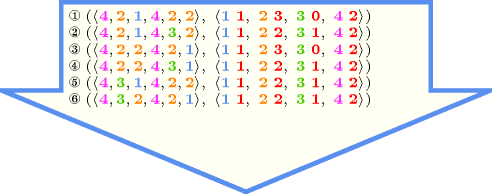Typical
 $|\mathrm{𝚅𝙰𝚁𝙸𝙰𝙱𝙻𝙴𝚂}|>1$ $\mathrm{𝚛𝚊𝚗𝚐𝚎}$$\left(\mathrm{𝚅𝙰𝚁𝙸𝙰𝙱𝙻𝙴𝚂}.\mathrm{𝚟𝚊𝚛}\right)>1$ $|\mathrm{𝚅𝙰𝙻𝚄𝙴𝚂}|>1$ $|\mathrm{𝚅𝙰𝚁𝙸𝙰𝙱𝙻𝙴𝚂}|\ge |\mathrm{𝚅𝙰𝙻𝚄𝙴𝚂}|$ $\mathrm{𝚖𝚒𝚗𝚟𝚊𝚕}$$\left(\mathrm{𝚅𝙰𝚁𝙸𝙰𝙱𝙻𝙴𝚂}.\mathrm{𝚟𝚊𝚛}\right)=0\vee$$\mathrm{𝚒𝚗}_\mathrm{𝚊𝚝𝚝𝚛}$$\left(\mathrm{𝚅𝙰𝚁𝙸𝙰𝙱𝙻𝙴𝚂},\mathrm{𝚟𝚊𝚛},\mathrm{𝚅𝙰𝙻𝚄𝙴𝚂},\mathrm{𝚟𝚊𝚕}\right)$
Symmetries
• Items of $\mathrm{𝚅𝙰𝚁𝙸𝙰𝙱𝙻𝙴𝚂}$ are permutable.

• Items of $\mathrm{𝚅𝙰𝙻𝚄𝙴𝚂}$ are permutable.

• An occurrence of a value of $\mathrm{𝚅𝙰𝚁𝙸𝙰𝙱𝙻𝙴𝚂}.\mathrm{𝚟𝚊𝚛}$ that does not belong to $\mathrm{𝚅𝙰𝙻𝚄𝙴𝚂}.\mathrm{𝚟𝚊𝚕}$ can be replaced by any other value that also does not belong to $\mathrm{𝚅𝙰𝙻𝚄𝙴𝚂}.\mathrm{𝚟𝚊𝚕}$.

• All occurrences of two distinct values in $\mathrm{𝚅𝙰𝚁𝙸𝙰𝙱𝙻𝙴𝚂}.\mathrm{𝚟𝚊𝚛}$ or $\mathrm{𝚅𝙰𝙻𝚄𝙴𝚂}.\mathrm{𝚟𝚊𝚕}$ can be swapped; all occurrences of a value in $\mathrm{𝚅𝙰𝚁𝙸𝙰𝙱𝙻𝙴𝚂}.\mathrm{𝚟𝚊𝚛}$ or $\mathrm{𝚅𝙰𝙻𝚄𝙴𝚂}.\mathrm{𝚟𝚊𝚕}$ can be renamed to any unused value.

Arg. properties
• Functional dependency: $\mathrm{𝚅𝙰𝙻𝚄𝙴𝚂}.\mathrm{𝚗𝚘𝚌𝚌𝚞𝚛𝚛𝚎𝚗𝚌𝚎}$ determined by $\mathrm{𝚅𝙰𝚁𝙸𝙰𝙱𝙻𝙴𝚂}$ and $\mathrm{𝚅𝙰𝙻𝚄𝙴𝚂}.\mathrm{𝚟𝚊𝚕}$.

• Contractible wrt. $\mathrm{𝚅𝙰𝙻𝚄𝙴𝚂}$.

Usage

We show how to use the $\mathrm{𝚐𝚕𝚘𝚋𝚊𝚕}_\mathrm{𝚌𝚊𝚛𝚍𝚒𝚗𝚊𝚕𝚒𝚝𝚢}$ constraint in order to model the magic series problem [VanHentenryck89] with a single $\mathrm{𝚐𝚕𝚘𝚋𝚊𝚕}_\mathrm{𝚌𝚊𝚛𝚍𝚒𝚗𝚊𝚕𝚒𝚝𝚢}$ constraint. A non-empty finite series $S=\left({s}_{0},{s}_{1},\cdots ,{s}_{n}\right)$ is magic if and only if there are ${s}_{i}$ occurrences of $i$ in $S$ for each integer $i$ ranging from 0 to $n$. This leads to the following model:

$\mathrm{𝚐𝚕𝚘𝚋𝚊𝚕}_\mathrm{𝚌𝚊𝚛𝚍𝚒𝚗𝚊𝚕𝚒𝚝𝚢}\left(\begin{array}{c}〈\begin{array}{c}\mathrm{𝚟𝚊𝚛}-{s}_{0},\mathrm{𝚟𝚊𝚛}-{s}_{1},\cdots ,\mathrm{𝚟𝚊𝚛}-{s}_{n}\hfill \end{array}〉,\hfill \\ 〈\begin{array}{cc}\mathrm{𝚟𝚊𝚕}-0\hfill & \mathrm{𝚗𝚘𝚌𝚌𝚞𝚛𝚛𝚎𝚗𝚌𝚎}-{s}_{0},\hfill \\ \mathrm{𝚟𝚊𝚕}-1\hfill & \mathrm{𝚗𝚘𝚌𝚌𝚞𝚛𝚛𝚎𝚗𝚌𝚎}-{s}_{1},\hfill \\ & ⋮\hfill \\ \mathrm{𝚟𝚊𝚕}-n\hfill & \mathrm{𝚗𝚘𝚌𝚌𝚞𝚛𝚛𝚎𝚗𝚌𝚎}-{s}_{n}\hfill \end{array}〉\hfill \end{array}\right)$
Remark

This is a generalised form of the original $\mathrm{𝚐𝚕𝚘𝚋𝚊𝚕}_\mathrm{𝚌𝚊𝚛𝚍𝚒𝚗𝚊𝚕𝚒𝚝𝚢}$ constraint: in the original $\mathrm{𝚐𝚕𝚘𝚋𝚊𝚕}_\mathrm{𝚌𝚊𝚛𝚍𝚒𝚗𝚊𝚕𝚒𝚝𝚢}$ constraint [Regin96], one specifies for each value its minimum and maximum number of occurrences (i.e., see $\mathrm{𝚐𝚕𝚘𝚋𝚊𝚕}_\mathrm{𝚌𝚊𝚛𝚍𝚒𝚗𝚊𝚕𝚒𝚝𝚢}_\mathrm{𝚕𝚘𝚠}_\mathrm{𝚞𝚙}$). Here we give for each value $v$ a domain variable that indicates how many time value $v$ is actually used. By setting the minimum and maximum values of this variable to the appropriate constants we can express the same thing as in the original $\mathrm{𝚐𝚕𝚘𝚋𝚊𝚕}_\mathrm{𝚌𝚊𝚛𝚍𝚒𝚗𝚊𝚕𝚒𝚝𝚢}$ constraint. However, as shown in the magic series problem, we can also use this variable in other constraints. By reduction from 3-SAT, Claude-Guy Quimper shows in [Quimper03] that it is NP-hard to achieve arc-consistency for the count variables.

A last difference with the original $\mathrm{𝚐𝚕𝚘𝚋𝚊𝚕}_\mathrm{𝚌𝚊𝚛𝚍𝚒𝚗𝚊𝚕𝚒𝚝𝚢}$ constraint comes from the fact that there is no constraint on the values that are not explicitly mentioned in the $\mathrm{𝚅𝙰𝙻𝚄𝙴𝚂}$ collection. In the original $\mathrm{𝚐𝚕𝚘𝚋𝚊𝚕}_\mathrm{𝚌𝚊𝚛𝚍𝚒𝚗𝚊𝚕𝚒𝚝𝚢}$ these values could not be assigned to the variables of the $\mathrm{𝚅𝙰𝚁𝙸𝙰𝙱𝙻𝙴𝚂}$ collection. However allowing values that are not mentioned in $\mathrm{𝚅𝙰𝙻𝚄𝙴𝚂}$ to be assigned to variables of $\mathrm{𝚅𝙰𝚁𝙸𝙰𝙱𝙻𝙴𝚂}$ can potentially avoid mentioning a huge number of unconstrained values in the $\mathrm{𝚅𝙰𝙻𝚄𝙴𝚂}$ collection, and as a side effect, prevent possiblyOf course one could also, while generating a flow model, detect all unconstrained values in order to generate a single vertex in the flow model for the set of unconstrained values. generating a dense graph (i.e., see DFS-bottleneck) for the corresponding underlying flow model).

Within [BourdaisGalinierPesant03] the $\mathrm{𝚐𝚕𝚘𝚋𝚊𝚕}_\mathrm{𝚌𝚊𝚛𝚍𝚒𝚗𝚊𝚕𝚒𝚝𝚢}$ constraint is called $\mathrm{𝚍𝚒𝚜𝚝𝚛𝚒𝚋𝚞𝚝𝚒𝚘𝚗}$. Within [ReginGomes04] the $\mathrm{𝚐𝚕𝚘𝚋𝚊𝚕}_\mathrm{𝚌𝚊𝚛𝚍𝚒𝚗𝚊𝚕𝚒𝚝𝚢}$ constraint is called $\mathrm{𝚌𝚊𝚛𝚍}_\mathrm{𝚟𝚊𝚛}_\mathrm{𝚐𝚌𝚌}$. Within [BessiereHebrardHnichWalsh04a] the $\mathrm{𝚐𝚕𝚘𝚋𝚊𝚕}_\mathrm{𝚌𝚊𝚛𝚍𝚒𝚗𝚊𝚕𝚒𝚝𝚢}$ constraint is called $\mathrm{𝚎𝚐𝚌𝚌}$ or $\mathrm{𝚛𝚐𝚌𝚌}$. This later case corresponds to the fact that some variables are duplicated within the $\mathrm{𝚅𝙰𝚁𝙸𝙰𝙱𝙻𝙴𝚂}$ collection.

The $\mathrm{𝚐𝚕𝚘𝚋𝚊𝚕}_\mathrm{𝚌𝚊𝚛𝚍𝚒𝚗𝚊𝚕𝚒𝚝𝚢}$ constraint can be seen as a system (i.e., a conjunction) of $\mathrm{𝚊𝚖𝚘𝚗𝚐}$ constraints.

When all count variables (i.e., the variables $\mathrm{𝚅𝙰𝙻𝚄𝙴𝚂}\left[i\right].\mathrm{𝚗𝚘𝚌𝚌𝚞𝚛𝚛𝚎𝚗𝚌𝚎}$ with $i\in \left[1,|\mathrm{𝚅𝙰𝙻𝚄𝙴𝚂}|\right]$) do not occur in any other constraints of the problem, it may be operationally more efficient to replace the $\mathrm{𝚐𝚕𝚘𝚋𝚊𝚕}_\mathrm{𝚌𝚊𝚛𝚍𝚒𝚗𝚊𝚕𝚒𝚝𝚢}$ constraint by a $\mathrm{𝚐𝚕𝚘𝚋𝚊𝚕}_\mathrm{𝚌𝚊𝚛𝚍𝚒𝚗𝚊𝚕𝚒𝚝𝚢}_\mathrm{𝚕𝚘𝚠}_\mathrm{𝚞𝚙}$ constraint where each count variable $\mathrm{𝚅𝙰𝙻𝚄𝙴𝚂}\left[i\right].\mathrm{𝚗𝚘𝚌𝚌𝚞𝚛𝚛𝚎𝚗𝚌𝚎}$ is replaced by the corresponding interval $\left[\underline{\mathrm{𝚅𝙰𝙻𝚄𝙴𝚂}\left[i\right].\mathrm{𝚗𝚘𝚌𝚌𝚞𝚛𝚛𝚎𝚗𝚌𝚎}},\overline{\mathrm{𝚅𝙰𝙻𝚄𝙴𝚂}\left[i\right].\mathrm{𝚗𝚘𝚌𝚌𝚞𝚛𝚛𝚎𝚗𝚌𝚎}}\right]$. This stands for two reasons:

When all values that can be assigned to the variables of the $\mathrm{𝚅𝙰𝚁𝙸𝙰𝙱𝙻𝙴𝚂}$ collection occur in the $\mathrm{𝚟𝚊𝚕}$ attribute of the $\mathrm{𝚅𝙰𝙻𝚄𝙴𝚂}$ collection, two implicit necessary conditionsNote that such necessary conditions can be derived by assigning an integer weight to each value [Simonis13], e.g. 1 for the first condition, the value itself for the second condition. inferred by double counting with the $\mathrm{𝚐𝚕𝚘𝚋𝚊𝚕}_\mathrm{𝚌𝚊𝚛𝚍𝚒𝚗𝚊𝚕𝚒𝚝𝚢}$ constraint are depicted by the following expressions:

$|\mathrm{𝚅𝙰𝚁𝙸𝙰𝙱𝙻𝙴𝚂}|=\sum _{i=1}^{|\mathrm{𝚅𝙰𝙻𝚄𝙴𝚂}|}\mathrm{𝚅𝙰𝙻𝚄𝙴𝚂}\left[i\right].\mathrm{𝚗𝚘𝚌𝚌𝚞𝚛𝚛𝚎𝚗𝚌𝚎}$
$\sum _{i=1}^{|\mathrm{𝚅𝙰𝚁𝙸𝙰𝙱𝙻𝙴𝚂}|}\mathrm{𝚅𝙰𝚁𝙸𝙰𝙱𝙻𝙴𝚂}\left[i\right].\mathrm{𝚟𝚊𝚛}=\sum _{i=1}^{|\mathrm{𝚅𝙰𝙻𝚄𝙴𝚂}|}\mathrm{𝚅𝙰𝙻𝚄𝙴𝚂}\left[i\right].\mathrm{𝚟𝚊𝚕}·\mathrm{𝚅𝙰𝙻𝚄𝙴𝚂}\left[i\right].\mathrm{𝚗𝚘𝚌𝚌𝚞𝚛𝚛𝚎𝚗𝚌𝚎}$

Within  [Pitrat08] the previous condition where terms involving identical variables are grouped together (i.e., rule 5 of MALICE [Pitrat01]) is mentioned as a crucial deduction rule for the autoref problem.

W.-J. van Hoeve et al. present two soft versions of the $\mathrm{𝚐𝚕𝚘𝚋𝚊𝚕}_\mathrm{𝚌𝚊𝚛𝚍𝚒𝚗𝚊𝚕𝚒𝚝𝚢}$ constraint in [HoevePesantRousseau04].

In MiniZinc (http://www.minizinc.org/) there is also a $\mathrm{𝚍𝚒𝚜𝚝𝚛𝚒𝚋𝚞𝚝𝚎}$ constraint where the $\mathrm{𝚟𝚊𝚕}$ attribute is not necessarily initially fixed and where a same value may occur more than once. Their is also a $\mathrm{𝚐𝚕𝚘𝚋𝚊𝚕}_\mathrm{𝚌𝚊𝚛𝚍𝚒𝚗𝚊𝚕𝚒𝚝𝚢}_\mathrm{𝚌𝚕𝚘𝚜𝚎𝚍}$ constraint where all variables must be assigned a value from the $\mathrm{𝚟𝚊𝚕}$ attribute.

Algorithm

A flow algorithm that handles the original $\mathrm{𝚐𝚕𝚘𝚋𝚊𝚕}_\mathrm{𝚌𝚊𝚛𝚍𝚒𝚗𝚊𝚕𝚒𝚝𝚢}$ constraint is described in [Regin96]. The two approaches that were used to design bound-consistency algorithms for $\mathrm{𝚊𝚕𝚕𝚍𝚒𝚏𝚏𝚎𝚛𝚎𝚗𝚝}$ were generalised for the $\mathrm{𝚐𝚕𝚘𝚋𝚊𝚕}_\mathrm{𝚌𝚊𝚛𝚍𝚒𝚗𝚊𝚕𝚒𝚝𝚢}$ constraint. The algorithm in [QuimperBeekOrtizGolynskiSadjad03] identifies Hall intervals and the one in [KatrielThiel03] exploits convexity to achieve a fast implementation of the flow-based arc-consistency algorithm. The later algorithm can also compute bound-consistency for the count variables [KatrielThielConstraints05], [Katriel05]. An improved algorithm for achieving arc-consistency is described in [QuimperLopezOrtizBeekGolynski04].

Systems

cost variant: $\mathrm{𝚐𝚕𝚘𝚋𝚊𝚕}_\mathrm{𝚌𝚊𝚛𝚍𝚒𝚗𝚊𝚕𝚒𝚝𝚢}_\mathrm{𝚠𝚒𝚝𝚑}_\mathrm{𝚌𝚘𝚜𝚝𝚜}$ ($\mathrm{𝚌𝚘𝚜𝚝}$ associated with each $\mathrm{𝚟𝚊𝚛𝚒𝚊𝚋𝚕𝚎}$,$\mathrm{𝚟𝚊𝚕𝚞𝚎}$ pair).

related: $\mathrm{𝚛𝚘𝚘𝚝𝚜}$, $\mathrm{𝚜𝚕𝚒𝚍𝚒𝚗𝚐}_\mathrm{𝚌𝚊𝚛𝚍}_\mathrm{𝚜𝚔𝚒𝚙}\mathtt{0}$ (counting constraint of a set of values on maximal sequences).

shift of concept: $\mathrm{𝚐𝚕𝚘𝚋𝚊𝚕}_\mathrm{𝚌𝚊𝚛𝚍𝚒𝚗𝚊𝚕𝚒𝚝𝚢}_\mathrm{𝚗𝚘}_\mathrm{𝚕𝚘𝚘𝚙}$ (assignment of a $\mathrm{𝚟𝚊𝚛𝚒𝚊𝚋𝚕𝚎}$ to its position is ignored), $\mathrm{𝚘𝚛𝚍𝚎𝚛𝚎𝚍}_\mathrm{𝚐𝚕𝚘𝚋𝚊𝚕}_\mathrm{𝚌𝚊𝚛𝚍𝚒𝚗𝚊𝚕𝚒𝚝𝚢}$ (restrictions are done on nested sets of values, all starting from first value), $\mathrm{𝚜𝚢𝚖𝚖𝚎𝚝𝚛𝚒𝚌}_\mathrm{𝚌𝚊𝚛𝚍𝚒𝚗𝚊𝚕𝚒𝚝𝚢}$, $\mathrm{𝚜𝚢𝚖𝚖𝚎𝚝𝚛𝚒𝚌}_\mathrm{𝚐𝚌𝚌}$.

soft variant: $\mathrm{𝚘𝚙𝚎𝚗}_\mathrm{𝚐𝚕𝚘𝚋𝚊𝚕}_\mathrm{𝚌𝚊𝚛𝚍𝚒𝚗𝚊𝚕𝚒𝚝𝚢}$ (a $\mathrm{𝚜𝚎𝚝}$ $\mathrm{𝚟𝚊𝚛𝚒𝚊𝚋𝚕𝚎}$ defines the set of variables that are actually considered).

specialisation: $\mathrm{𝚊𝚕𝚕𝚍𝚒𝚏𝚏𝚎𝚛𝚎𝚗𝚝}$ (each value should occur at most once), $\mathrm{𝚌𝚊𝚛𝚍𝚒𝚗𝚊𝚕𝚒𝚝𝚢}_\mathrm{𝚊𝚝𝚕𝚎𝚊𝚜𝚝}$, $\mathrm{𝚌𝚊𝚛𝚍𝚒𝚗𝚊𝚕𝚒𝚝𝚢}_\mathrm{𝚊𝚝𝚖𝚘𝚜𝚝}$ (individual $\mathrm{𝚌𝚘𝚞𝚗𝚝}\mathrm{𝚟𝚊𝚛𝚒𝚊𝚋𝚕𝚎}$ for each value replaced by single $\mathrm{𝚌𝚘𝚞𝚗𝚝}\mathrm{𝚟𝚊𝚛𝚒𝚊𝚋𝚕𝚎}$), $\mathrm{𝚌𝚊𝚛𝚍𝚒𝚗𝚊𝚕𝚒𝚝𝚢}_\mathrm{𝚊𝚝𝚖𝚘𝚜𝚝}_\mathrm{𝚙𝚊𝚛𝚝𝚒𝚝𝚒𝚘𝚗}$ (individual $\mathrm{𝚌𝚘𝚞𝚗𝚝}\mathrm{𝚟𝚊𝚛𝚒𝚊𝚋𝚕𝚎}$ for each value replaced by single $\mathrm{𝚌𝚘𝚞𝚗𝚝}\mathrm{𝚟𝚊𝚛𝚒𝚊𝚋𝚕𝚎}$ and $\mathrm{𝚟𝚊𝚛𝚒𝚊𝚋𝚕𝚎}\in \mathrm{𝚙𝚊𝚛𝚝𝚒𝚝𝚒𝚘𝚗}$ replaced by $\mathrm{𝚟𝚊𝚛𝚒𝚊𝚋𝚕𝚎}$), $\mathrm{𝚐𝚕𝚘𝚋𝚊𝚕}_\mathrm{𝚌𝚊𝚛𝚍𝚒𝚗𝚊𝚕𝚒𝚝𝚢}_\mathrm{𝚕𝚘𝚠}_\mathrm{𝚞𝚙}$ ($\mathrm{𝚟𝚊𝚛𝚒𝚊𝚋𝚕𝚎}$ replaced by $\mathrm{𝚏𝚒𝚡𝚎𝚍}$ $\mathrm{𝚒𝚗𝚝𝚎𝚛𝚟𝚊𝚕}$).

system of constraints: $\mathrm{𝚌𝚘𝚕𝚘𝚛𝚎𝚍}_\mathrm{𝚖𝚊𝚝𝚛𝚒𝚡}$ (one $\mathrm{𝚐𝚕𝚘𝚋𝚊𝚕}_\mathrm{𝚌𝚊𝚛𝚍𝚒𝚗𝚊𝚕𝚒𝚝𝚢}$ constraint for each $\mathrm{𝚛𝚘𝚠}$ and each $\mathrm{𝚌𝚘𝚕𝚞𝚖𝚗}$ of a $\mathrm{𝚖𝚊𝚝𝚛𝚒𝚡}$ of $\mathrm{𝚟𝚊𝚛𝚒𝚊𝚋𝚕𝚎𝚜}$).

Keywords
Cond. implications

$•$ $\mathrm{𝚐𝚕𝚘𝚋𝚊𝚕}_\mathrm{𝚌𝚊𝚛𝚍𝚒𝚗𝚊𝚕𝚒𝚝𝚢}\left(\mathrm{𝚅𝙰𝚁𝙸𝙰𝙱𝙻𝙴𝚂},\mathrm{𝚅𝙰𝙻𝚄𝙴𝚂}\right)$

with  $\mathrm{𝚖𝚒𝚗𝚟𝚊𝚕}$$\left(\mathrm{𝚅𝙰𝚁𝙸𝙰𝙱𝙻𝙴𝚂}.\mathrm{𝚟𝚊𝚛}\right)=0$

implies $\mathrm{𝚊𝚗𝚍}$$\left(\mathrm{𝚅𝙰𝚁},\mathrm{𝚅𝙰𝚁𝙸𝙰𝙱𝙻𝙴𝚂}\right)$

when  $\mathrm{𝚅𝙰𝚁}=0$.

$•$ $\mathrm{𝚐𝚕𝚘𝚋𝚊𝚕}_\mathrm{𝚌𝚊𝚛𝚍𝚒𝚗𝚊𝚕𝚒𝚝𝚢}\left(\mathrm{𝚅𝙰𝚁𝙸𝙰𝙱𝙻𝙴𝚂},\mathrm{𝚅𝙰𝙻𝚄𝙴𝚂}\right)$

with  $\mathrm{𝚖𝚊𝚡𝚟𝚊𝚕}$$\left(\mathrm{𝚅𝙰𝚁𝙸𝙰𝙱𝙻𝙴𝚂}.\mathrm{𝚟𝚊𝚛}\right)=1$

implies $\mathrm{𝚘𝚛}$$\left(\mathrm{𝚅𝙰𝚁},\mathrm{𝚅𝙰𝚁𝙸𝙰𝙱𝙻𝙴𝚂}\right)$

when  $\mathrm{𝚅𝙰𝚁}=1$.

$•$ $\mathrm{𝚐𝚕𝚘𝚋𝚊𝚕}_\mathrm{𝚌𝚊𝚛𝚍𝚒𝚗𝚊𝚕𝚒𝚝𝚢}\left(\mathrm{𝚅𝙰𝚁𝙸𝙰𝙱𝙻𝙴𝚂},\mathrm{𝚅𝙰𝙻𝚄𝙴𝚂}\right)$

with  $\mathrm{𝚖𝚒𝚗𝚟𝚊𝚕}$$\left(\mathrm{𝚅𝙰𝚁𝙸𝙰𝙱𝙻𝙴𝚂}.\mathrm{𝚟𝚊𝚛}\right)>0$

implies $\mathrm{𝚖𝚒𝚗}_\mathrm{𝚜𝚒𝚣𝚎}_\mathrm{𝚏𝚞𝚕𝚕}_\mathrm{𝚣𝚎𝚛𝚘}_\mathrm{𝚜𝚝𝚛𝚎𝚝𝚌𝚑}$$\left(\mathrm{𝙼𝙸𝙽𝚂𝙸𝚉𝙴},\mathrm{𝚅𝙰𝚁𝙸𝙰𝙱𝙻𝙴𝚂}\right)$

when  $\mathrm{𝙼𝙸𝙽𝚂𝙸𝚉𝙴}=|\mathrm{𝚅𝙰𝚁𝙸𝙰𝙱𝙻𝙴𝚂}|$.

$•$ $\mathrm{𝚐𝚕𝚘𝚋𝚊𝚕}_\mathrm{𝚌𝚊𝚛𝚍𝚒𝚗𝚊𝚕𝚒𝚝𝚢}\left(\mathrm{𝚅𝙰𝚁𝙸𝙰𝙱𝙻𝙴𝚂},\mathrm{𝚅𝙰𝙻𝚄𝙴𝚂}\right)$

with  $\mathrm{𝚖𝚊𝚡𝚟𝚊𝚕}$$\left(\mathrm{𝚅𝙰𝚁𝙸𝙰𝙱𝙻𝙴𝚂}.\mathrm{𝚟𝚊𝚛}\right)<0$

implies $\mathrm{𝚖𝚒𝚗}_\mathrm{𝚜𝚒𝚣𝚎}_\mathrm{𝚏𝚞𝚕𝚕}_\mathrm{𝚣𝚎𝚛𝚘}_\mathrm{𝚜𝚝𝚛𝚎𝚝𝚌𝚑}$$\left(\mathrm{𝙼𝙸𝙽𝚂𝙸𝚉𝙴},\mathrm{𝚅𝙰𝚁𝙸𝙰𝙱𝙻𝙴𝚂}\right)$

when  $\mathrm{𝙼𝙸𝙽𝚂𝙸𝚉𝙴}=|\mathrm{𝚅𝙰𝚁𝙸𝙰𝙱𝙻𝙴𝚂}|$.

$•$ $\mathrm{𝚐𝚕𝚘𝚋𝚊𝚕}_\mathrm{𝚌𝚊𝚛𝚍𝚒𝚗𝚊𝚕𝚒𝚝𝚢}\left(\mathrm{𝚅𝙰𝚁𝙸𝙰𝙱𝙻𝙴𝚂},\mathrm{𝚅𝙰𝙻𝚄𝙴𝚂}\right)$

with  $\mathrm{𝚛𝚊𝚗𝚐𝚎}$$\left(\mathrm{𝚅𝙰𝙻𝚄𝙴𝚂}.\mathrm{𝚟𝚊𝚕}\right)=$$\mathrm{𝚗𝚟𝚊𝚕}$$\left(\mathrm{𝚅𝙰𝙻𝚄𝙴𝚂}.\mathrm{𝚟𝚊𝚕}\right)$

and   $\mathrm{𝚖𝚒𝚗𝚟𝚊𝚕}$$\left(\mathrm{𝚅𝙰𝙻𝚄𝙴𝚂}.\mathrm{𝚟𝚊𝚕}\right)\le$$\mathrm{𝚖𝚒𝚗𝚟𝚊𝚕}$$\left(\mathrm{𝚅𝙰𝚁𝙸𝙰𝙱𝙻𝙴𝚂}.\mathrm{𝚟𝚊𝚛}\right)$

and   $\mathrm{𝚖𝚊𝚡𝚟𝚊𝚕}$$\left(\mathrm{𝚅𝙰𝙻𝚄𝙴𝚂}.\mathrm{𝚟𝚊𝚕}\right)\ge$$\mathrm{𝚖𝚊𝚡𝚟𝚊𝚕}$$\left(\mathrm{𝚅𝙰𝚁𝙸𝙰𝙱𝙻𝙴𝚂}.\mathrm{𝚟𝚊𝚛}\right)$

implies $\mathrm{𝚊𝚖𝚘𝚗𝚐}_\mathrm{𝚍𝚒𝚏𝚏}_\mathtt{0}$$\left(\mathrm{𝙽𝚅𝙰𝚁},\mathrm{𝚅𝙰𝚁𝙸𝙰𝙱𝙻𝙴𝚂}\right)$.

$•$ $\mathrm{𝚐𝚕𝚘𝚋𝚊𝚕}_\mathrm{𝚌𝚊𝚛𝚍𝚒𝚗𝚊𝚕𝚒𝚝𝚢}\left(\mathrm{𝚅𝙰𝚁𝙸𝙰𝙱𝙻𝙴𝚂},\mathrm{𝚅𝙰𝙻𝚄𝙴𝚂}\right)$

with  $\mathrm{𝚛𝚊𝚗𝚐𝚎}$$\left(\mathrm{𝚅𝙰𝙻𝚄𝙴𝚂}.\mathrm{𝚟𝚊𝚕}\right)=$$\mathrm{𝚗𝚟𝚊𝚕}$$\left(\mathrm{𝚅𝙰𝙻𝚄𝙴𝚂}.\mathrm{𝚟𝚊𝚕}\right)$

and   $\mathrm{𝚖𝚒𝚗𝚟𝚊𝚕}$$\left(\mathrm{𝚅𝙰𝙻𝚄𝙴𝚂}.\mathrm{𝚟𝚊𝚕}\right)\le$$\mathrm{𝚖𝚒𝚗𝚟𝚊𝚕}$$\left(\mathrm{𝚅𝙰𝚁𝙸𝙰𝙱𝙻𝙴𝚂}.\mathrm{𝚟𝚊𝚛}\right)$

and   $\mathrm{𝚖𝚊𝚡𝚟𝚊𝚕}$$\left(\mathrm{𝚅𝙰𝙻𝚄𝙴𝚂}.\mathrm{𝚟𝚊𝚕}\right)\ge$$\mathrm{𝚖𝚊𝚡𝚟𝚊𝚕}$$\left(\mathrm{𝚅𝙰𝚁𝙸𝙰𝙱𝙻𝙴𝚂}.\mathrm{𝚟𝚊𝚛}\right)$

implies $\mathrm{𝚊𝚝𝚖𝚘𝚜𝚝}_\mathrm{𝚗𝚟𝚊𝚕𝚞𝚎}$$\left(\mathrm{𝙽𝚅𝙰𝙻},\mathrm{𝚅𝙰𝚁𝙸𝙰𝙱𝙻𝙴𝚂}\right)$.

$•$ $\mathrm{𝚐𝚕𝚘𝚋𝚊𝚕}_\mathrm{𝚌𝚊𝚛𝚍𝚒𝚗𝚊𝚕𝚒𝚝𝚢}\left(\mathrm{𝚅𝙰𝚁𝙸𝙰𝙱𝙻𝙴𝚂},\mathrm{𝚅𝙰𝙻𝚄𝙴𝚂}\right)$

with  $\mathrm{𝚛𝚊𝚗𝚐𝚎}$$\left(\mathrm{𝚅𝙰𝙻𝚄𝙴𝚂}.\mathrm{𝚗𝚘𝚌𝚌𝚞𝚛𝚛𝚎𝚗𝚌𝚎}\right)=1$

and   $\mathrm{𝚛𝚊𝚗𝚐𝚎}$$\left(\mathrm{𝚅𝙰𝙻𝚄𝙴𝚂}.\mathrm{𝚟𝚊𝚕}\right)=$$\mathrm{𝚗𝚟𝚊𝚕}$$\left(\mathrm{𝚅𝙰𝙻𝚄𝙴𝚂}.\mathrm{𝚟𝚊𝚕}\right)$

and   $\mathrm{𝚖𝚒𝚗𝚟𝚊𝚕}$$\left(\mathrm{𝚅𝙰𝙻𝚄𝙴𝚂}.\mathrm{𝚟𝚊𝚕}\right)=$$\mathrm{𝚖𝚒𝚗𝚟𝚊𝚕}$$\left(\mathrm{𝚅𝙰𝚁𝙸𝙰𝙱𝙻𝙴𝚂}.\mathrm{𝚟𝚊𝚛}\right)$

and   $\mathrm{𝚖𝚊𝚡𝚟𝚊𝚕}$$\left(\mathrm{𝚅𝙰𝙻𝚄𝙴𝚂}.\mathrm{𝚟𝚊𝚕}\right)=$$\mathrm{𝚖𝚊𝚡𝚟𝚊𝚕}$$\left(\mathrm{𝚅𝙰𝚁𝙸𝙰𝙱𝙻𝙴𝚂}.\mathrm{𝚟𝚊𝚛}\right)$

implies $\mathrm{𝚋𝚊𝚕𝚊𝚗𝚌𝚎}$$\left(\mathrm{𝙱𝙰𝙻𝙰𝙽𝙲𝙴},\mathrm{𝚅𝙰𝚁𝙸𝙰𝙱𝙻𝙴𝚂}\right)$

when  $\mathrm{𝙱𝙰𝙻𝙰𝙽𝙲𝙴}=0$.

$•$ $\mathrm{𝚐𝚕𝚘𝚋𝚊𝚕}_\mathrm{𝚌𝚊𝚛𝚍𝚒𝚗𝚊𝚕𝚒𝚝𝚢}\left(\mathrm{𝚅𝙰𝚁𝙸𝙰𝙱𝙻𝙴𝚂},\mathrm{𝚅𝙰𝙻𝚄𝙴𝚂}\right)$

with  $\mathrm{𝚛𝚊𝚗𝚐𝚎}$$\left(\mathrm{𝚅𝙰𝙻𝚄𝙴𝚂}.\mathrm{𝚟𝚊𝚕}\right)=$$\mathrm{𝚗𝚟𝚊𝚕}$$\left(\mathrm{𝚅𝙰𝙻𝚄𝙴𝚂}.\mathrm{𝚟𝚊𝚕}\right)$

and   $\mathrm{𝚖𝚒𝚗𝚟𝚊𝚕}$$\left(\mathrm{𝚅𝙰𝙻𝚄𝙴𝚂}.\mathrm{𝚟𝚊𝚕}\right)\le$$\mathrm{𝚖𝚒𝚗𝚟𝚊𝚕}$$\left(\mathrm{𝚅𝙰𝚁𝙸𝙰𝙱𝙻𝙴𝚂}.\mathrm{𝚟𝚊𝚛}\right)$

and   $\mathrm{𝚖𝚊𝚡𝚟𝚊𝚕}$$\left(\mathrm{𝚅𝙰𝙻𝚄𝙴𝚂}.\mathrm{𝚟𝚊𝚕}\right)\ge$$\mathrm{𝚖𝚊𝚡𝚟𝚊𝚕}$$\left(\mathrm{𝚅𝙰𝚁𝙸𝙰𝙱𝙻𝙴𝚂}.\mathrm{𝚟𝚊𝚛}\right)$

implies $\mathrm{𝚖𝚊𝚡}_𝚗$$\left(\mathrm{𝙼𝙰𝚇},\mathrm{𝚁𝙰𝙽𝙺},\mathrm{𝚅𝙰𝚁𝙸𝙰𝙱𝙻𝙴𝚂}\right)$.

$•$ $\mathrm{𝚐𝚕𝚘𝚋𝚊𝚕}_\mathrm{𝚌𝚊𝚛𝚍𝚒𝚗𝚊𝚕𝚒𝚝𝚢}\left(\mathrm{𝚅𝙰𝚁𝙸𝙰𝙱𝙻𝙴𝚂},\mathrm{𝚅𝙰𝙻𝚄𝙴𝚂}\right)$

with  $\mathrm{𝚛𝚊𝚗𝚐𝚎}$$\left(\mathrm{𝚅𝙰𝙻𝚄𝙴𝚂}.\mathrm{𝚟𝚊𝚕}\right)=$$\mathrm{𝚗𝚟𝚊𝚕}$$\left(\mathrm{𝚅𝙰𝙻𝚄𝙴𝚂}.\mathrm{𝚟𝚊𝚕}\right)$

and   $\mathrm{𝚖𝚒𝚗𝚟𝚊𝚕}$$\left(\mathrm{𝚅𝙰𝙻𝚄𝙴𝚂}.\mathrm{𝚟𝚊𝚕}\right)\le$$\mathrm{𝚖𝚒𝚗𝚟𝚊𝚕}$$\left(\mathrm{𝚅𝙰𝚁𝙸𝙰𝙱𝙻𝙴𝚂}.\mathrm{𝚟𝚊𝚛}\right)$

and   $\mathrm{𝚖𝚊𝚡𝚟𝚊𝚕}$$\left(\mathrm{𝚅𝙰𝙻𝚄𝙴𝚂}.\mathrm{𝚟𝚊𝚕}\right)\ge$$\mathrm{𝚖𝚊𝚡𝚟𝚊𝚕}$$\left(\mathrm{𝚅𝙰𝚁𝙸𝙰𝙱𝙻𝙴𝚂}.\mathrm{𝚟𝚊𝚛}\right)$

implies $\mathrm{𝚖𝚊𝚡}_\mathrm{𝚗𝚟𝚊𝚕𝚞𝚎}$$\left(\mathrm{𝙼𝙰𝚇},\mathrm{𝚅𝙰𝚁𝙸𝙰𝙱𝙻𝙴𝚂}\right)$.

$•$ $\mathrm{𝚐𝚕𝚘𝚋𝚊𝚕}_\mathrm{𝚌𝚊𝚛𝚍𝚒𝚗𝚊𝚕𝚒𝚝𝚢}\left(\mathrm{𝚅𝙰𝚁𝙸𝙰𝙱𝙻𝙴𝚂},\mathrm{𝚅𝙰𝙻𝚄𝙴𝚂}\right)$

with  $\mathrm{𝚛𝚊𝚗𝚐𝚎}$$\left(\mathrm{𝚅𝙰𝙻𝚄𝙴𝚂}.\mathrm{𝚟𝚊𝚕}\right)=$$\mathrm{𝚗𝚟𝚊𝚕}$$\left(\mathrm{𝚅𝙰𝙻𝚄𝙴𝚂}.\mathrm{𝚟𝚊𝚕}\right)$

and   $\mathrm{𝚖𝚒𝚗𝚟𝚊𝚕}$$\left(\mathrm{𝚅𝙰𝙻𝚄𝙴𝚂}.\mathrm{𝚟𝚊𝚕}\right)\le$$\mathrm{𝚖𝚒𝚗𝚟𝚊𝚕}$$\left(\mathrm{𝚅𝙰𝚁𝙸𝙰𝙱𝙻𝙴𝚂}.\mathrm{𝚟𝚊𝚛}\right)$

and   $\mathrm{𝚖𝚊𝚡𝚟𝚊𝚕}$$\left(\mathrm{𝚅𝙰𝙻𝚄𝙴𝚂}.\mathrm{𝚟𝚊𝚕}\right)\ge$$\mathrm{𝚖𝚊𝚡𝚟𝚊𝚕}$$\left(\mathrm{𝚅𝙰𝚁𝙸𝙰𝙱𝙻𝙴𝚂}.\mathrm{𝚟𝚊𝚛}\right)$

implies $\mathrm{𝚖𝚒𝚗}_𝚗$$\left(\mathrm{𝙼𝙸𝙽},\mathrm{𝚁𝙰𝙽𝙺},\mathrm{𝚅𝙰𝚁𝙸𝙰𝙱𝙻𝙴𝚂}\right)$.

$•$ $\mathrm{𝚐𝚕𝚘𝚋𝚊𝚕}_\mathrm{𝚌𝚊𝚛𝚍𝚒𝚗𝚊𝚕𝚒𝚝𝚢}\left(\mathrm{𝚅𝙰𝚁𝙸𝙰𝙱𝙻𝙴𝚂},\mathrm{𝚅𝙰𝙻𝚄𝙴𝚂}\right)$

with  $\mathrm{𝚛𝚊𝚗𝚐𝚎}$$\left(\mathrm{𝚅𝙰𝙻𝚄𝙴𝚂}.\mathrm{𝚟𝚊𝚕}\right)=$$\mathrm{𝚗𝚟𝚊𝚕}$$\left(\mathrm{𝚅𝙰𝙻𝚄𝙴𝚂}.\mathrm{𝚟𝚊𝚕}\right)$

and   $\mathrm{𝚖𝚒𝚗𝚟𝚊𝚕}$$\left(\mathrm{𝚅𝙰𝙻𝚄𝙴𝚂}.\mathrm{𝚟𝚊𝚕}\right)\le$$\mathrm{𝚖𝚒𝚗𝚟𝚊𝚕}$$\left(\mathrm{𝚅𝙰𝚁𝙸𝙰𝙱𝙻𝙴𝚂}.\mathrm{𝚟𝚊𝚛}\right)$

and   $\mathrm{𝚖𝚊𝚡𝚟𝚊𝚕}$$\left(\mathrm{𝚅𝙰𝙻𝚄𝙴𝚂}.\mathrm{𝚟𝚊𝚕}\right)\ge$$\mathrm{𝚖𝚊𝚡𝚟𝚊𝚕}$$\left(\mathrm{𝚅𝙰𝚁𝙸𝙰𝙱𝙻𝙴𝚂}.\mathrm{𝚟𝚊𝚛}\right)$

implies $\mathrm{𝚖𝚒𝚗}_\mathrm{𝚗𝚟𝚊𝚕𝚞𝚎}$$\left(\mathrm{𝙼𝙸𝙽},\mathrm{𝚅𝙰𝚁𝙸𝙰𝙱𝙻𝙴𝚂}\right)$.

$•$ $\mathrm{𝚐𝚕𝚘𝚋𝚊𝚕}_\mathrm{𝚌𝚊𝚛𝚍𝚒𝚗𝚊𝚕𝚒𝚝𝚢}\left(\mathrm{𝚅𝙰𝚁𝙸𝙰𝙱𝙻𝙴𝚂},\mathrm{𝚅𝙰𝙻𝚄𝙴𝚂}\right)$

with  $\mathrm{𝚛𝚊𝚗𝚐𝚎}$$\left(\mathrm{𝚅𝙰𝙻𝚄𝙴𝚂}.\mathrm{𝚟𝚊𝚕}\right)=$$\mathrm{𝚗𝚟𝚊𝚕}$$\left(\mathrm{𝚅𝙰𝙻𝚄𝙴𝚂}.\mathrm{𝚟𝚊𝚕}\right)$

and   $\mathrm{𝚖𝚒𝚗𝚟𝚊𝚕}$$\left(\mathrm{𝚅𝙰𝙻𝚄𝙴𝚂}.\mathrm{𝚟𝚊𝚕}\right)\le$$\mathrm{𝚖𝚒𝚗𝚟𝚊𝚕}$$\left(\mathrm{𝚅𝙰𝚁𝙸𝙰𝙱𝙻𝙴𝚂}.\mathrm{𝚟𝚊𝚛}\right)$

and   $\mathrm{𝚖𝚊𝚡𝚟𝚊𝚕}$$\left(\mathrm{𝚅𝙰𝙻𝚄𝙴𝚂}.\mathrm{𝚟𝚊𝚕}\right)\ge$$\mathrm{𝚖𝚊𝚡𝚟𝚊𝚕}$$\left(\mathrm{𝚅𝙰𝚁𝙸𝙰𝙱𝙻𝙴𝚂}.\mathrm{𝚟𝚊𝚛}\right)$

implies $\mathrm{𝚛𝚊𝚗𝚐𝚎}_\mathrm{𝚌𝚝𝚛}$$\left(\mathrm{𝚅𝙰𝚁𝙸𝙰𝙱𝙻𝙴𝚂},\mathrm{𝙲𝚃𝚁},𝚁\right)$.

For all items of $\mathrm{𝚅𝙰𝙻𝚄𝙴𝚂}$:

Arc input(s)

$\mathrm{𝚅𝙰𝚁𝙸𝙰𝙱𝙻𝙴𝚂}$

Arc generator
$\mathrm{𝑆𝐸𝐿𝐹}$$↦\mathrm{𝚌𝚘𝚕𝚕𝚎𝚌𝚝𝚒𝚘𝚗}\left(\mathrm{𝚟𝚊𝚛𝚒𝚊𝚋𝚕𝚎𝚜}\right)$

Arc arity
Arc constraint(s)
$\mathrm{𝚟𝚊𝚛𝚒𝚊𝚋𝚕𝚎𝚜}.\mathrm{𝚟𝚊𝚛}=\mathrm{𝚅𝙰𝙻𝚄𝙴𝚂}.\mathrm{𝚟𝚊𝚕}$
Graph property(ies)
$\mathrm{𝐍𝐕𝐄𝐑𝐓𝐄𝐗}$$=\mathrm{𝚅𝙰𝙻𝚄𝙴𝚂}.\mathrm{𝚗𝚘𝚌𝚌𝚞𝚛𝚛𝚎𝚗𝚌𝚎}$

Graph model

Since we want to express one unary constraint for each value we use the “For all items of $\mathrm{𝚅𝙰𝙻𝚄𝙴𝚂}$” iterator. Part (A) of Figure 5.163.2 shows the initial graphs associated with each value 3, 5 and 6 of the $\mathrm{𝚅𝙰𝙻𝚄𝙴𝚂}$ collection of the Example slot. Part (B) of Figure 5.163.2 shows the two corresponding final graphs respectively associated with values 3 and 6 that are both assigned to the variables of the $\mathrm{𝚅𝙰𝚁𝙸𝙰𝙱𝙻𝙴𝚂}$ collection (since value 5 is not assigned to any variable of the $\mathrm{𝚅𝙰𝚁𝙸𝙰𝙱𝙻𝙴𝚂}$ collection the final graph associated with value 5 is empty). Since we use the $\mathrm{𝐍𝐕𝐄𝐑𝐓𝐄𝐗}$ graph property, the vertices of the final graphs are stressed in bold.

##### Figure 5.163.2. Initial and final graph of the $\mathrm{𝚐𝚕𝚘𝚋𝚊𝚕}_\mathrm{𝚌𝚊𝚛𝚍𝚒𝚗𝚊𝚕𝚒𝚝𝚢}$ constraint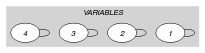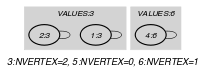(a) (b)
Automaton

Figure 5.163.3 depicts the automaton associated with the $\mathrm{𝚐𝚕𝚘𝚋𝚊𝚕}_\mathrm{𝚌𝚊𝚛𝚍𝚒𝚗𝚊𝚕𝚒𝚝𝚢}$ constraint. To each item of the collection $\mathrm{𝚅𝙰𝚁𝙸𝙰𝙱𝙻𝙴𝚂}$ corresponds a signature variable ${S}_{i}$ that is equal to 0. To each item of the collection $\mathrm{𝚅𝙰𝙻𝚄𝙴𝚂}$ corresponds a signature variable ${S}_{i+|\mathrm{𝚅𝙰𝚁𝙸𝙰𝙱𝙻𝙴𝚂}|}$ that is equal to 1.

##### Figure 5.163.3. Automaton of the $\mathrm{𝚐𝚕𝚘𝚋𝚊𝚕}_\mathrm{𝚌𝚊𝚛𝚍𝚒𝚗𝚊𝚕𝚒𝚝𝚢}$ constraint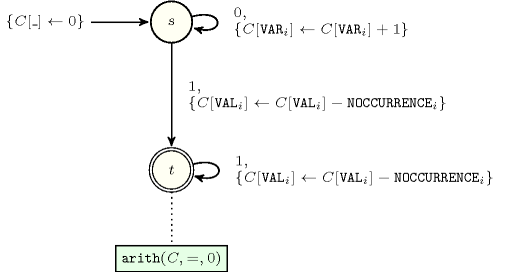Quiz

$\mathrm{𝚐𝚕𝚘𝚋𝚊𝚕}_\mathrm{𝚌𝚊𝚛𝚍𝚒𝚗𝚊𝚕𝚒𝚝𝚢}$: checking whether a ground instance holds or not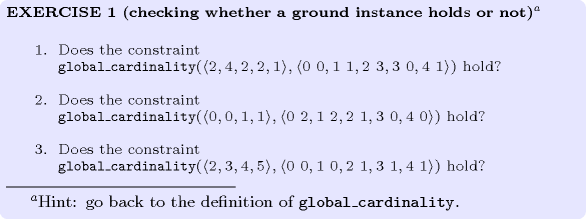$\mathrm{𝚐𝚕𝚘𝚋𝚊𝚕}_\mathrm{𝚌𝚊𝚛𝚍𝚒𝚗𝚊𝚕𝚒𝚝𝚢}$: finding all solutions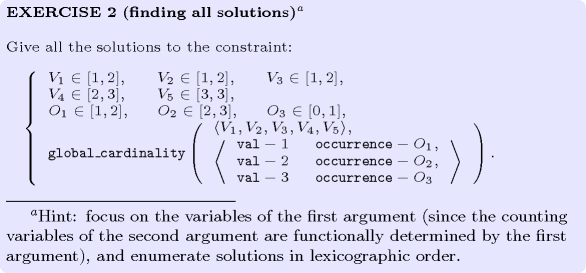$\mathrm{𝚐𝚕𝚘𝚋𝚊𝚕}_\mathrm{𝚌𝚊𝚛𝚍𝚒𝚗𝚊𝚕𝚒𝚝𝚢}$: identifying infeasible values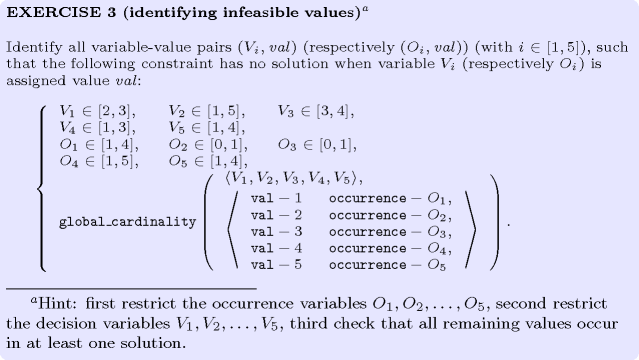$\mathrm{𝚐𝚕𝚘𝚋𝚊𝚕}_\mathrm{𝚌𝚊𝚛𝚍𝚒𝚗𝚊𝚕𝚒𝚝𝚢}$: modelling a nurse assignment problem